الفصل الأول

# مراجعة الوحدة الثانية حاسوب صف عاشر متقدم فصل أول

## مراجعة الوحدة الثانية حاسوب صف عاشر متقدم فصل أول

مرفق لكم مراجعة الوحدة الثانية حاسوب صف عاشر متقدم فصل أول مناهج الامارات

### معلومات المذكرة

• نوع الملف: ملف مراجعة
• المادة: حاسوب
• الصف: العاشر
• الفصل الدراسي: الفصل الاول
• صيغة الملف: pptx متاح للتحميل

### صندوق تحميل الملف

What is the
? conditional statements

Conditional statements are expressions that give either a true or false result

Simple conditional statements

an expression that uses relational operators to compare values; they evaluate to either true or false
emanAge > aishaAge

Compound conditional statements

an expression that combines multiple simple conditional statements using logical operators
(emanAge > aishaAge)and (emanAge == monaAge)

? What is relational operators
operators used to compare values such as

? What is logical operators
operators used to evaluate expressions which result in either true or false such as

? What is If statement
Selection/decision statement used to choose the code blocks to run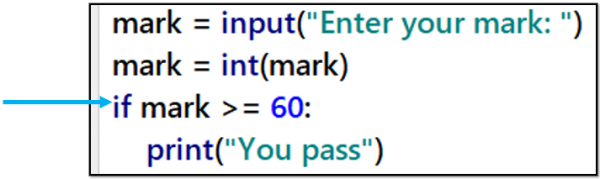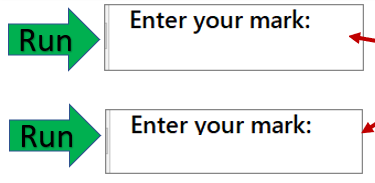? How can you make the code work with 2 condition

The if-else statement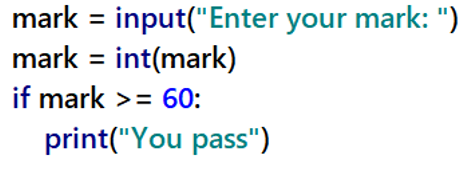bottleA = input(“Enter the volume of bottle A: “)
bottleA = int(bottleA)

bottleB = input(“Enter the volume of bottle B: “)
bottleB = int(bottleB)

if bottleA > bottleB:
print(“Bottle A is bigger.”)
else:
print(“Bottle B is bigger.”)

number = input(“Enter the number: “)
number = int(number)

if number >0
print (“The number is positive.”)
else:
print (“The number is negative.”)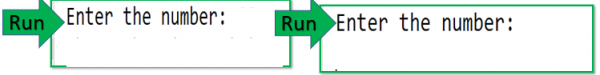? What if the user input 0

Run : Enter the number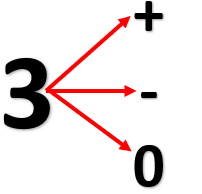? How many options must in the program

if you have more than two conditions
أكثر من حالتين؟The elf statement

Design an algorithm to determine the development stage of people based on their age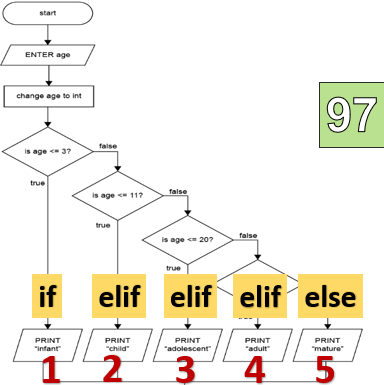bottleA = input(“Enter the volume of bottle A: “)
bottleA = int(bottleA)

bottleB = input(“Enter the volume of bottle B: “)
bottleB = int(bottleB)

if bottleA > bottleB:
print(“Bottle A is bigger.”)
else:
print(“Bottle B is bigger.”)

? What would happen if they have the same volume
Another condition is needed

bottleA = input(“Enter the volume of bottle A: “)
bottleA = int(bottleA)

bottleB = input(“Enter the volume of bottle B: “)
bottleB = int(bottleB)

if bottleA > bottleB:
print(“Bottle A is bigger.”)
elif bottleA > bottleB:
print(“Bottle B is bigger.”)
else:
print(“Bottle A and B have the same volume.”)

a) Improve your program from activity 22(c) to cover conditions when the bolt is smaller than  7.5mm, or if an invalid diameter, such as a negative number is entered.

boltDiameter = input(“Enter the diameter of the bolt: “)
boltDiameter = float(boltDiameter)

if boltDiameter > 7.5:
print(“Reject. The bolt is too big.”)
else:
print(“Accept.”)

elif boltDiameter > 0:
print(“Accept.”)
else:
print(“Invalid number”)

b) Design a Python program to print a comment based on a student’s mark. The user should enter the marks. Test your code for marks from every range.

Mark range Less than 0 or greater than 100
Mark range Greater than or equal to 0 and less than 40
Try harder next time
Mark range Greater than or equal to 40 and less than 50
You can do better
Mark range Greater than or equal to 50 and less than 75
Very good
Mark range Greater than or equal to 75 and less than or equal to 100
Excellent

d) Design a program to tell a drinks factory manager about the level of the orange juice in the tank. Your program should check and print a message for the following cases

There is enough juice when the tank has more than 210 liters •
The tank is about to run out of juice when the tank has between 15 and 60 liters left •
It should also say when the tank gets empty •

? Is there a quick way to write if statement

print(“Pass”)
else:
print(“Fail”)

result = “Pass” if (grade >= 60) else “Fail
print(result)

The conditional operator

Is a quick way to write if statements to set a variable to a value you want based on the result of a conditional statement.

result = “Pass” if (grade >= 60) else “Fail”
print(result)

Exception handling

A user entering a data type or value into a program which the programmer did not think of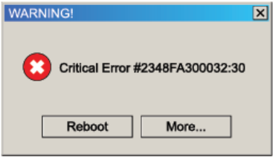They can cause the program to crash

mass = input(“Enter the mass: “)
mass = int(mass)
volume = input(“Enter the volume: “)
volume = int(volume)

The program will crash

density = mass / volume
print(“Density = “, density, “Kg/m3”)

? What happened if the input of volume is 0

mass = input(“Enter the mass: “)
mass = int(mass)
volume = input(“Enter the volume: “)
volume = int(volume)

The program will crash again!

if volume > 0:
density = mass / volume
print(“Density = “, density, “Kg/m3”)
else:
print(“Error: cannot divide by zero volume”)

mass = input(“Enter the mass: “)
mass = int(mass)
volume = input(“Enter the volume: “)
volume = int(volume)

try:
density = mass / volume
print(“Density = “, density, “Kg/m3”)
except:
print(“Error: cannot divide by zero volume.”)

You can use the try and except block for any code which could create errors

Exception handling
Code blocks used to handle unexpected error conditions while the program is running.

Repetition
The statements used to repeat code are called loops

while loop
تكرار لعدد غير معلوم من المرات

for loop
تكرار لعدد معلوم من المرات

While loops

Repeat the same code block for as long as the result of a conditional statement remains true. The number of times the code block is repeated is unknown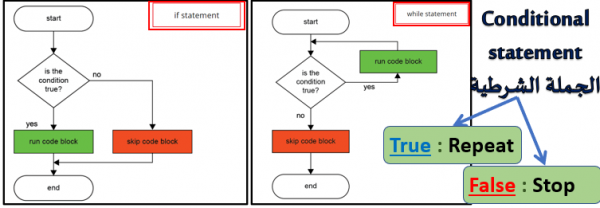people = 0

while people < 30:
morePeople = input(“How many more people? “)
morePeople = int(morePeople)
people = people + morePeople

print(“There are “, people, ” people on the train”)

30 times
30 times

The simple conditional statement evaluates to false when people = 30

a)  Run the code and enter 1 each time you are asked to enter a number
? How many times are you asked to enter a number •
? How many times is the while loop executed •
? Why did it stop •

b) Now enter 5 each time you are asked to enter a number.
? How many times are you asked to enter a number •
? How many times is the while loop executed •
? Why did it stop •

6 times
6 times
The simple conditional statement evaluates to false when people = 30.

c) Now enter 7.
? How many times are you asked to enter a number this time •
? How many times did the while loop run •
? Why did it stop •

5 times
5 times
The simple conditional statement evaluates to false when people > 30.

تصفح أيضا: